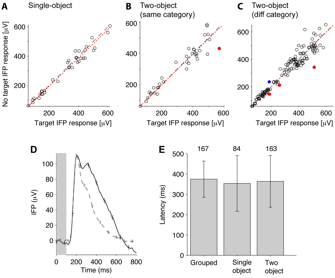Robustness to two-object images in human visual cortex

Figure W5: Comparison of responses to target-present images versus target-absent imagesA-C. For each visually selective electrode, we compare the responses to the preferred object category in images where the preferred category was the target (x-axis) and images where the target category was absent. The response is the IFP range from 50 to 300 ms after stimulus onset. The dotted line is the linear fit to the data and is very close to the diagonal (dashed line). Red (blue) circles denote cases where the response to target images was significantly greater (smaller) than the response to non-target images (two-tailed t-test, p<0.05). A. Single-object images. B. Images containing two objects from the same category. C. Images containing two objects from different categories. D. Example electrode showing late differences between target present and target absent trials. This electrode was located in the left inferior temporal cortex. The plot shows the average responses to all images where the target category was present (solid black line) and all the images where the target category was not present (dashed gray line). Error bars denote one SEM. Note that the solid and dashed lines start to separate around 300 ms after stimulus onset. E. Latencies for discriminating images that contained the target from images that did not contain the target. At each time point, we performed a t-test between the IFP for target images and non-target images. The latency was defined as the first time point where n=15 time points yielded p<0.01 in this analysis. The number of electrodes that passed this criterion is indicated above each bar. “Grouped” refers to all images. “Single object” refers to images containing single objects. “Two-object” refers to images containing two objects from different categories. Error bars denote one SD.

Top